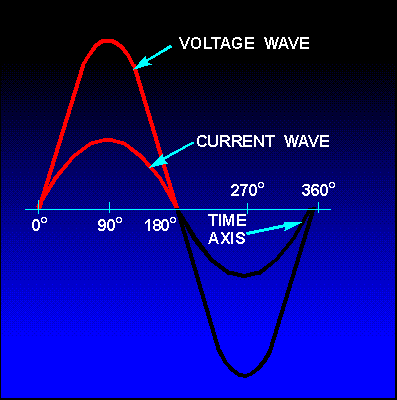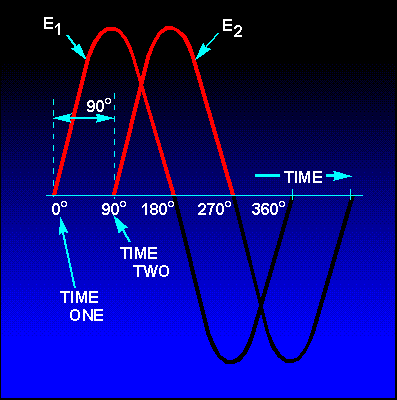resistance, the resulting current is also a sine wave. This follows Ohm's law which states that current is directly proportional to the applied voltage. Now examine figure 1-17. Notice that the sine wave of voltage and the resulting sine wave of current are superimposed on the same time axis. Notice also that as the voltage increases in a positive direction, the current increases along with it, and that when the voltage reverses direction, the current also reverses direction.">Custom SearchSINE WAVES IN PHASE When a sine wave of voltage is applied to a resistance, the resulting current is also a sine wave. This follows Ohm's law which states that current is directly proportional to the applied voltage. Now examine figure 1-17. Notice that the sine wave of voltage and the resulting sine wave of current are superimposed on the same time axis. Notice also that as the voltage increases in a positive direction, the current increases along with it, and that when the voltage reverses direction, the current also reverses direction. When two sine waves, such as those represented by figure 1-17, are precisely in step with one another, they are said to be IN PHASE. To be in phase, the two sine waves must go through their maximum and minimum points at the same time and in the same direction. Figure 1-17. - Voltage and current waves in phase.In some circuits, several sine waves can be in phase with each other. Thus, it is possible to have two or more voltage drops in phase with each other and also be in phase with the circuit current. SINE WAVES OUT OF PHASE Figure 1-18 shows voltage wave E 1 which is considered to start at 0 (time one). As voltage wave E1 reaches its positive peak, voltage wave E 2 starts its rise (time two). Since these voltage waves do not go through their maximum and minimum points at the same instant of time, a PHASE DIFFERENCE exists between the two waves. The two waves are said to be OUT OF PHASE. For the two waves in figure 1-18 the phase difference is 90. Figure 1-18. - Voltage waves 90 out of phase.To further describe the phase relationship between two sine waves, the terms LEAD and LAG are used. The amount by which one sine wave leads or lags another sine wave is measured in degrees. Refer again to figure 1-18. Observe that wave E2 starts 90 later in time than does wave E1. You can also describe this relationship by saying that wave E1 leads wave E 2 by 90, or that wave E2 lags wave E 1 by 90. (Either statement is correct; it is the phase relationship between the two sine waves that is important.) It is possible for one sine wave to lead or lag another sine wave by any number of degrees, except 0 or 360. When the latter condition exists, the two waves are said to be in phase. Thus, two sine waves that differ in phase by 45 are actually out of phase with each other, whereas two sine waves that differ in phase by 360 are considered to be in phase with each other. A phase relationship that is quite common is shown in figure 1-19. Notice that the two waves illustrated differ in phase by 180. Notice also that although the waves pass through their maximum and minimum values at the same time, their instantaneous voltages are always of opposite polarity. If two such waves exist across the same component, and the waves are of equal amplitude, they cancel each other. When they have different amplitudes, the resultant wave has the same polarity as the larger wave and has an amplitude equal to the difference between the amplitudes of the two waves. Figure 1-19. - Voltage waves 180 out of phase.To determine the phase difference between two sine waves, locate the points on the time axis where the two waves cross the time axis traveling in the same direction. The number of degrees between the crossing points is the phase difference. The wave that crosses the axis at the later time (to the right on the time axis) is said to lag the other wave. Q.37 When are the voltage wave and the current wave in a circuit considered to be in phase? Q.38 When are two voltage waves considered to be out of phase? Q.39 What is the phase relationship between two voltage waves that differ in phase by 360 ? Q.40 How do you determine the phase difference between two sine waves that are plotted on the same graph?Integrated Publishing, Inc. - A (SDVOSB) Service Disabled Veteran Owned Small Business